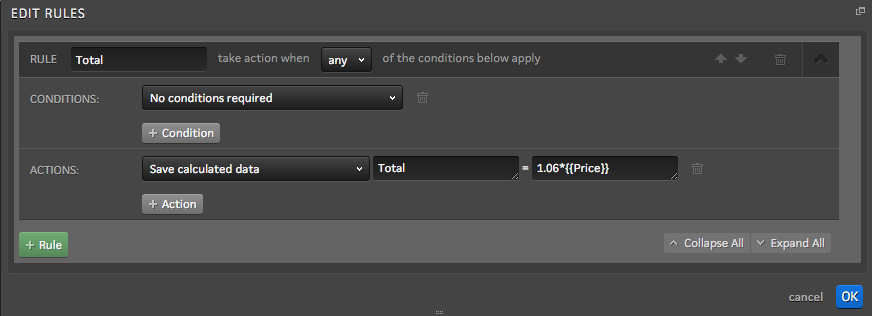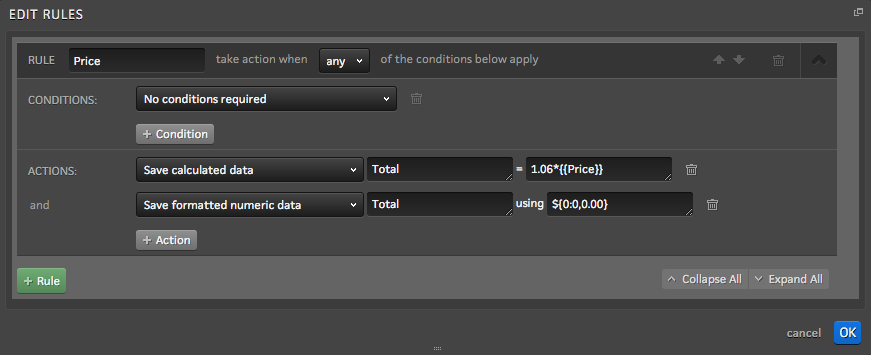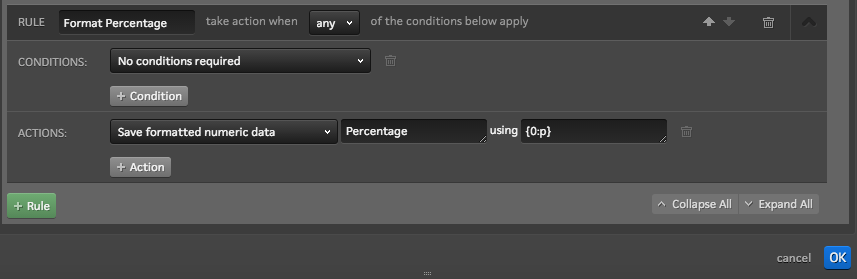# Save Formatted Numeric Data

## When setting up advanced rules, you may find yourself in a situation where you need to format the data differently than what is returned as the value of your calculation.

For example, you may want to set up a calculator experience that shows visitors how much something would cost after sales tax is applied to it. If you want to do this for a specific state, you could fix the sales tax percentage and set up a calculation rule on your form

Let’s say our form is set up with a data field in it called “Price.” Let’s also use 6% for sales tax. You could add a rule to your form that would look like the below to multiply the price by 1.06 (1.06*{{Price}} ) to find the total which would be saved into a data field called “Total.”In this example, the total often times would have more than two digits to the right of the decimal and would not include any commas when something is more than \$999.

The “Save formatted numeric data” action can be used to include commas and to ensure only two digits are present to the right of the decimal point. This action can be added to the original rule as seen below.In this example, the Save formatted numeric data action will take the total that was calculated in the action above it and ensure that it only has two digits to the right of the decimal. If more than two digits are returned from the calculation, this action will truncate the decimal to only include two digits and will round up or down based on the third digit to the right of the decimal. This action will also add a comma for every three digits to the left of the decimal (i.e. 1,000) and will save a dollar sign in front of the total.

If you would like to format a dollar amount without decimals, you could use the \${0:0,0} format.

If someone were to enter 1523.73 into the form field, the total price with sales tax that would be saved would be \$1,615.15 as opposed to 1615.1538. You could also display this total on your Thank You page using dynamic content insertion.

#### Standard Numeric Formats

There are also more standardized formats that can be set up with the format numeric data action. For example, if you want to set up an experience that could convert a percentage that is calculated and format it accordingly, you could use the {0:p} format. Please note that for this rule would be based on the premise that we have a percentage already saved to the “Percent” field and we want to format it accordingly.• Also check our support article: Save computed data for more information on setting up rules to calculate values in Ion.
• To understand standard numeric formats that are available, click here .